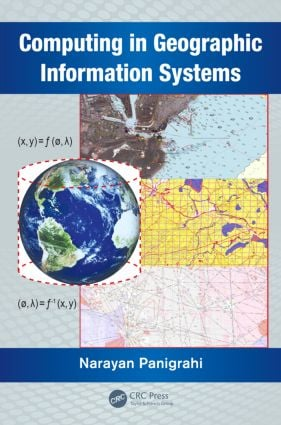# Computing in Geographic Information Systems

## 1st Edition

CRC Press

303 pages | 72 B/W Illus.

##### Purchasing Options:\$ = USD
Hardback: 9781482223149
pub: 2014-07-10
\$130.00
x
eBook (VitalSource) : 9780429069635
pub: 2014-07-10
from \$28.98

FREE Standard Shipping!

### Description

Capable of acquiring large volumes of data through sensors deployed in air, land, and sea, and making this information readily available in a continuous time frame, the science of geographical information system (GIS) is rapidly evolving. This popular information system is emerging as a platform for scientific visualization, simulation, and computation of spatio-temporal data. New computing techniques are being researched and implemented to match the increasing capability of modern-day computing platforms and easy availability of spatio-temporal data. This has led to the need for the design, analysis, development, and optimization of new algorithms for extracting spatio-temporal patterns from a large volume of spatial data.

Computing in Geographic Information Systems considers the computational aspects, and helps students understand the mathematical principles of GIS. It provides a deeper understanding of the algorithms and mathematical methods inherent in the process of designing and developing GIS functions. It examines the associated scientific computations along with the applications of computational geometry, differential geometry, and affine geometry in processing spatial data. It also covers the mathematical aspects of geodesy, cartography, map projection, spatial interpolation, spatial statistics, and coordinate transformation. The book discusses the principles of bathymetry and generation of electronic navigation charts.

The book consists of 12 chapters. Chapters one through four delve into the modeling and preprocessing of spatial data and prepares the spatial data as input to the GIS system. Chapters five through eight describe the various techniques of computing the spatial data using different geometric and statically techniques. Chapters nine through eleven define the technique for image registration computation and measurements of spatial objects and phenomenon.

• Examines cartographic modeling and map projection
• Covers the mathematical aspects of different map projections
• Explores some of the spatial analysis techniques and applications of GIS
• Introduces the bathymetric principles and systems generated using bathymetric charts
• Explains concepts of differential geometry, affine geometry, and computational geometry
• Discusses popular analysis and measurement methods used in GIS

This text outlines the key concepts encompassing GIS and spatio-temporal information, and is intended for students, researchers, and professionals engaged in analysis, visualization, and estimation of spatio-temporal events.

### Reviews

"This book addresses mathematics and computational methods that are not usually covered by common GIS books. Unlike other books that only teach people how to apply GIS and use GIS software, this book describes the underlying principles and mathematical equations. Engineers who develop innovative tools for GIS users would find this book useful."

––Shipeng Sun, University of Minnesota, Minneapolis, USA

"There are only few books that deal with mathematical aspects of GIS. This book will help to fill this gap. The most valuable contribution of this book is the fact that it effectively pervades mathematical equations and formulas to discuss geospatial concepts and principles. GIS topics are amply covered, and are approached in a way that will help students of GIS to gain greater knowledge of the computational aspects of spatial data collection, transformation, manipulation, analysis and application."

––Shrinidhi Ambinakudige, Mississippi State University

Introduction

Definitions and Different Perspectives of GIS

Computational Aspects of GIS

Computing Algorithms in GIS

Motivation of the Book

Organization of the Book

Summary

Computational Geodesy

Definition of Geodesy

Mathematical Models of Earth

Geometry of Ellipse and Ellipsoid

Computing Radius of Curvature

Concept of Latitude

Applications of Geodesy

The Indian Geodetic Reference System (IGRS)

Summary

Reference Systems and Coordinate Transformations

Definition of Reference System

Classification of Reference Systems

Datum and Coordinate System

Attachment of Datum to the Real World

Different Coordinate Systems Used in GIS

Shape of Earth

Coordinate Transformations

Datum Transformation

Usage of Coordinate Systems

Summary

Basics of Map Projection

What is Map Projection? and Why it is Necessary?

Mathematical Definition of Map Projection

Process Flow of Map Projection

Azimuthal Map Projection

Cylinderical Map Projection

Conical Map Projection

Classification of Map Projections

Application of Map Projections

Summary

Algorithms for Rectification of Geometric Distortions

Sources of Geometric Distortion

Algorithms for Satellite Image Registration

Scale Invariant Feature Transform (SIFT)

Fourier Mellin Transform

Multiresolution Image Analysis

Applications of Image Registration

Summary

Differential Geometric Principles and Operators

Properties of Gaussian, Hessian and Difference of Gaussian

Summary

Computational Geometry and its Application to GIS

Introduction

Definitions

Geometric Computational Techniques

Triangulation of Simple Polygon

Convex Hulls in Two Dimensions

Divide and Conquer Algorithm

Voronoi Diagrams

Delaunay Triangulation

Delaunay Triangulation: Randomized Incremental Algorithm

Delaunay Triangulations and Convex Hulls

Applications of Voronoi Diagram and Delaunay Triangulation

Summary

Spatial Interpolation Techniques

Non-Geostatistical Interpolators

Geostatistics

Summary

Spatial Statistical Methods

Definition of Statistics

Spatial statistics

Classification of statistical methods

Role of statistics in GIS

Descriptive Statistical Methods

Inferential Statistics

Point Pattern Analysis in GIS

Applications of Spatial Statistical Methods

Summary

An Introduction to Bathymetry

Spatial Analysis of Bathymetric Data and Sea GIS

Measurements and Analysis Using GIS

Appendix

Appendix-B

Glossary of GIS Terms

Bibliography

### About the Author

Dr. Narayan Panigrahi is a practicing geo-spatial scientist in the Centre for Artificial Intelligence and Robotics (CAIR), Bangalore, India. He obtained his master's degree from the J.K. Institute of Applied Physics and Technology, University of Allahabad, his M Tech from the Indian Institute of Technology (IIT), Kharagpur, and his PhD from IIT, Bombay, India. His current research interests include geographical information science, design and optimization of algorithms for computation of spatial data in vector and raster form obtained through different sensors, and application of GIS for resource mapping and operation planning.

### Subject Categories

##### BISAC Subject Codes/Headings:
MAT000000
MATHEMATICS / General
MAT003000
MATHEMATICS / Applied
TEC036000
TECHNOLOGY & ENGINEERING / Remote Sensing & Geographic Information Systems